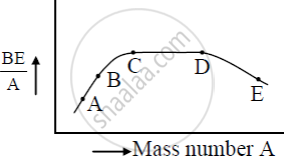# The Figure Shows the Plot of Binding Energy (Be) per Nucleon as a Function of Mass Number A. the Letters A, B, C, D, and E Represent the Positions of Typical Nuclei on the Curve. - Physics

Short Note

The figure shows the plot of binding energy (BE) per nucleon as a function of mass number A. The letters A, B, C, D, and E represent the positions of typical nuclei on the curve. Point out, giving reasons, the two processes (in terms of A, B, C, D, and E ), one of which can occur due to nuclear fission and the other due to nuclear fusion.#### Solution

The nuclei at A and B undergo nuclear fusion as their binding energy per nucleon is small and they are less stable so they fuse with other nuclei to become stable. The nuclei at E undergo nuclear fission as its binding energy per nucleon is less it splits into two or more lighter nuclei and becomes stable.

Concept: Mass-energy and Nuclear Binding Energy - Nuclear Binding Energy
Is there an error in this question or solution?